9I Quick Quiz

9Ia

1       Which of these is not a form of energy?

A       kinetic                            B       petrol

C       light                                D      sound

2       Which of these has the most gravitational potential energy?

A       a parachutist standing on a runway

B       a parachutist going into a plane

C       a parachutist jumping out of a plane

D       a parachutist after landing

3       What are the energy changes in a candle?

A       heat to light

B       heat to sound

C       light to heat

D       chemical to heat and light

4       A machine is something which:

A       changes energy.

B       creates energy.

C       destroys energy.

D       makes things.

9Ib

1       The units for voltage are:

A       amps.

B       ohms.

C       volts.

D       metres.

2       Voltage is measured:

 A         using a voltmeter in the circuit like this.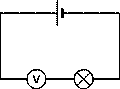B         using a voltmeter in the circuit like this.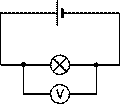C         using an ammeter in the circuit like this.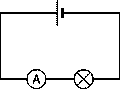D         using an ammeter in the circuit like this.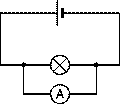3       Voltage is:

A       another name for current.

B       a way of counting how many cells there are in a circuit.

C       a way of saying how much energy the electricity is carrying.

D       something that makes it difficult for electricity to flow.

4       Which statement is not true for a series circuit?

A       The voltage is always the same for all the components.

B       The voltage across each component depends on how much energy it uses.

C       The voltage across all the components adds up to the voltage across the cell.

D       The voltage can be measured using a voltmeter.

9Ic

1       Where is electricity generated?

A       in the home                     B       in a power station

C       in the mains                     D      in the wires

2       The energy in electricity comes from:

A       energy stored in fuels, winds or water.

B       energy stored in food.

C       energy in the wires.

D       energy in the switches.

3       A cell is:

A       a jail.

B       a store of chemical energy.

C       mains electricity.

D       a power pack.

4       High voltages are dangerous because:

A       they cost a lot to produce.

B       they do not transfer much energy.

C       they can transfer a lot of energy.

D       they are poisonous.

9Id

1       Which statement is true?

A       You always put more energy into a machine than you get out.

B       You always get more energy out of a machine than you put in.

C       The amount of energy you get out of a machine is always exactly the same as the energy you put in.

D       The amount of energy you get out of a machine does not depend on the amount you put in.

2       Which is the correct energy flow diagram for a television?

 A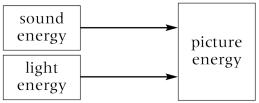B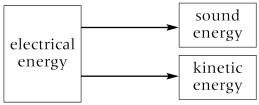C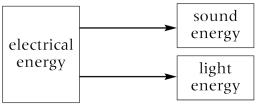D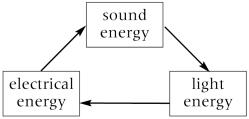3       Which forms of energy are often produced as wasted energy?

A       heat and light

B       heat and sound

C       sound and light

D       sound and kinetic

4       An efficient machine:

A       does not waste much energy.

B       wastes a lot of energy.

C       is more expensive to run than an inefficient machine.

D       is more expensive to buy than an inefficient machine.